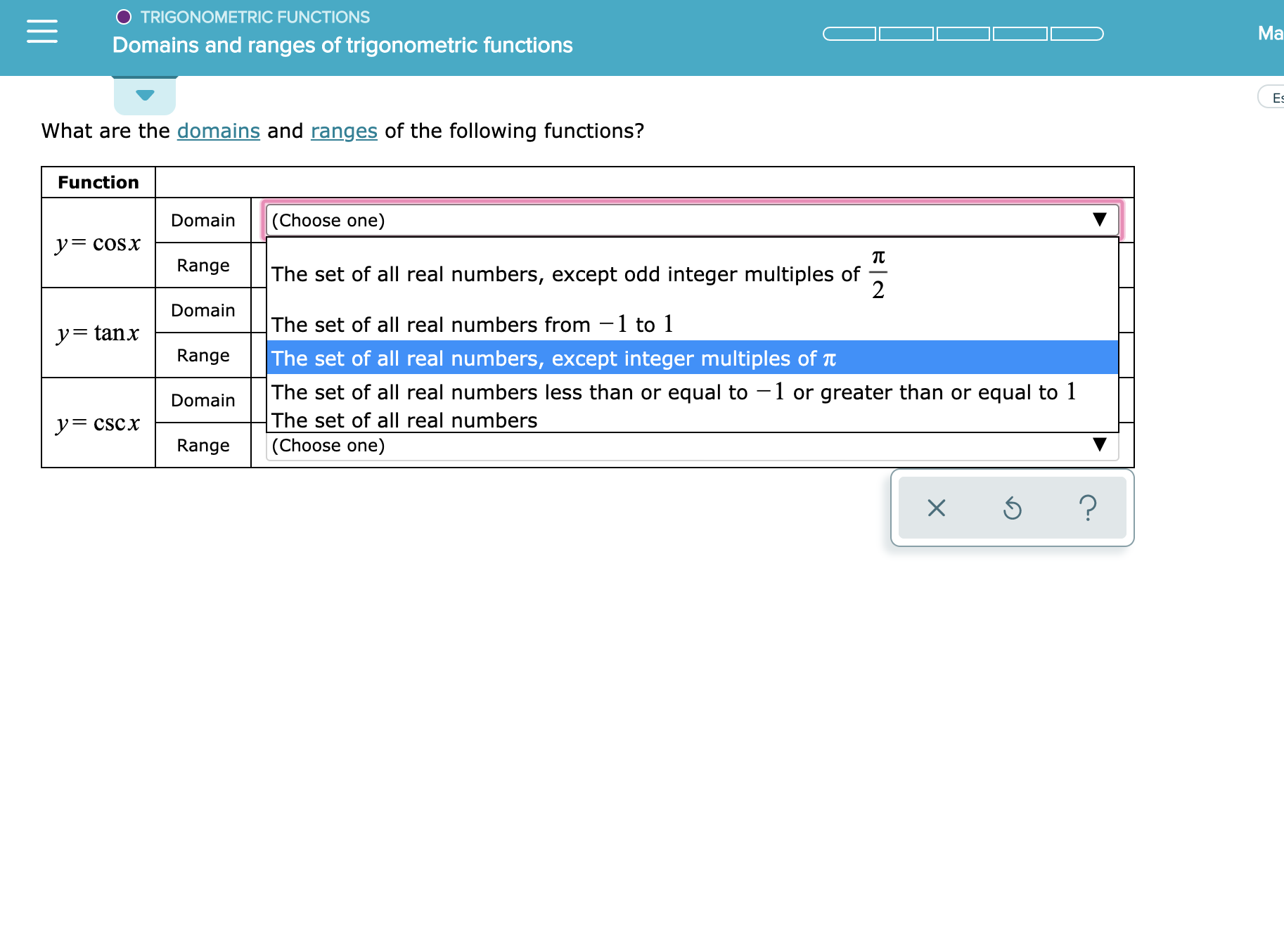# TRIGONOMETRIC FUNCTIONSMaDomains and ranges of trigonometric functionsEsWhat are the domains and ranges of the following functions?Function(Choose one)DomainycoSxRangeThe set of all real numbers, except odd integer multiples of2DomainThe set of all real numbers from -1 to 1y=tanxThe set of all real numbers, except integer multiples of tRangeThe set of all real numbers less than or equal to - 1 or greater than or equal to 1The set of all real numbersDomainyCSCx(Choose one)Range?X

Question
1 views

See attachmenthelp_outlineImage TranscriptioncloseTRIGONOMETRIC FUNCTIONS Ma Domains and ranges of trigonometric functions Es What are the domains and ranges of the following functions? Function (Choose one) Domain ycoSx Range The set of all real numbers, except odd integer multiples of 2 Domain The set of all real numbers from -1 to 1 y=tanx The set of all real numbers, except integer multiples of t Range The set of all real numbers less than or equal to - 1 or greater than or equal to 1 The set of all real numbers Domain yCSCx (Choose one) Range ? X fullscreen
check_circle

Step 1

Calculation:

Compute the domain and the range of the function  y = cos(x)  as follows.

It is known that the value of cos(x) lies between −1 and 1 for all real values of x.

Thus, the domain of y = cos(x) is the set of all real numbers.

Range of y = cos(x) is set of all real numbers from −1 to1.

Compute the domain and the range of the function  y = tan(x)  as follows.

Rewrite y = tan(x) as y = sin(x)/cos(x).

Since cos(0) = 0, the function is undefined for all integer multiplies of π and for 0.

Thus, the domain of y = cot(x) is the set of all real numbers ...

### Want to see the full answer?

See Solution

#### Want to see this answer and more?

Solutions are written by subject experts who are available 24/7. Questions are typically answered within 1 hour.*

See Solution
*Response times may vary by subject and question.
Tagged in

### Calculus